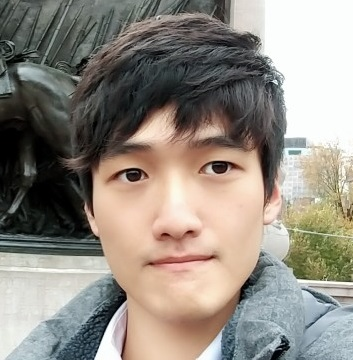Jaewoo Song

### Categories

• Tech

Attention mechasnism is one of the most important concepts in NLP field.

First, this mechanism itself was introduced in other fields, such as Computer Vision.

Then it have adapted into NLP models and ideas, which have enabled the progress of NLP research a lot.

Most of well-performing models these days, such as BERT, GPT etc. which are based on Transformer’s architecture, exist thanks to the advent of attention mechanism.

The attention mechanism in a seq2seq model was introduced in Bahdanau, D., Cho, K., & Bengio, Y. (2014). Neural machine translation by jointly learning to align and translate. arXiv preprint arXiv:1409.0473.

It was adapted to handle several problems from the limitations of original seq2seq model.

The problems are as follows.

• Since the whole input is compacted into one small context vector after the encoder, the loss of overall contexts cannot be avoided.
• Because a seq2seq model consists of RNN based models, it has original problems occurring from a basic RNN, such as the vanishing gradient problem. With LSTM or GRU, we can improve the RNN’s performance but these variations can solve the problems to some extent, but not very well.

Above issues eventually degrade the performance of the seq2seq model.

Therefore the attention mechanism came out to support it.

As we can notice from its name, the attention mechanism’s principle is that the decoder pays attention to encoder cells while making an output in decoding steps.

That is, when creating the result from the decoder, it can make better output by referring to the information in the input sequence in the encoder.

Let’s go back to the machine translation task last post.

The basic idea of attention is described like this.Attention function

Attention mechanism operates under certain function rules.

This function consists of three components, query, key, and value.

In seq2seq model, each component is as follows if we decode the token at time $t$.

• $Q$(Query): a decoder hidden state at time step $t-1$.
• $K$(Key): all encoder hidden states
• $V$(Value): all encoder hidden states

To put it simply, query is a question from current decoder cell which the model wants to make an output from.

The attention function calculates all similarities between current decoder hidden state(which is query) and all encoder hidden states(which is key).

Value is a final value from attention function based on these similarities.

The sum of these values are finally adapted to the decoder and helps it to make a suitable output.

The process of dot product attention in seq2seq model

Now, let’s see how the attention mechanism operates specifically.

There are several kinds of attention, but we are going to see the “dot product attention” which is the most commonly used.

Let’s assume that we want to make the third token “tokC” in the decoding process.

This is the process of dot product attention described.This procedure can be divided into 3 phases.

Let’s see that step by step.

1. Attention scoreFirst, we conduct the dot product between the decoder hidden state at the previous time step $t-1$($Q$) and all encoder hidden states($K$).

In this case, the query becomes the $2$nd hidden vector of the decoder.

Since the dot product is calculated with two vectors in the same size, it produces a scalar value.

Therefore, if we say that the length of input sequence is $L$ and the hidden size is $h$, then this phase conducts dot products between two $h$ dimensional vectors and produces $L$ scalar values.

These values are called “attention scores”.

2. Attention value (Context vector)We have $L$ attention scores which are put into the softmax layer.

By the softmax, these scores are regularized as values between $0$ and $1$, which indicate importance of each input token when making decoder output at the current time step.

So these attention weights are considered as we decide which input context(encoder hidden state) should be focused.

Now each encoder hidden state($V$) are multiplied with each attention weight and added up.

This vector after above weighted sum has the same size with encoder hidden state, which is $h$, and is called “attention value”.

Since it has overall context of which input context should be referred to make proper output from decoder cell at time $t$, it is also called “context vector”. (It should not be confused with the context vector which is the last hidden state from the encoder…)

3. Next decoder cell & next wordFinally, this attention value(context vector) and the original input to the next decoder cell(the word generated at time $t-1$) are concatenated into one vector.

This vector becomes the input to the next decoder cell.

So the decoder hidden states get this additional information from the attention mechanism in the decoding process, which leads to better results.

So this is the attention mechanism in seq2seq model.

As I mentioned before, the attention mechanism is used in various model besides the seq2seq model.

In the next post, we will see how the attention is adapted into simple RNN based model, not the seq2seq model.

Bahdanau, D., Cho, K., & Bengio, Y. (2014). Neural machine translation by jointly learning to align and translate. https://arxiv.org/abs/1409.0473
1) 어텐션 메커니즘 (Attention Mechanism). (2020, Feb 18). https://wikidocs.net/22893.